×#### Thank you for registering.

One of our academic counsellors will contact you within 1 working day.

Click to Chat

1800-1023-196

+91-120-4616500

CART 0

• 0

MY CART (5)

Use Coupon: CART20 and get 20% off on all online Study Material

ITEM
DETAILS
MRP
DISCOUNT
FINAL PRICE
Total Price: Rs.

There are no items in this cart.
Continue Shopping• Complete JEE Main/Advanced Course and Test Series
• OFFERED PRICE: Rs. 15,900
• View Details

```Chapter 13: Probability Exercise – 13.2

Question: 1

Suppose you drop a tie at random on the rectangular region shown in fig. below. What is the probability that it will land inside the circle with diameter 1 m?Solution:

Area of a circle with radius 0.5 m A circle = (0.5)2 = 0.25 πm2

Area of rectangle = 3 x 2 = 6m2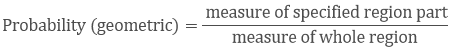The probability that tie will land inside the circle with diameter 1mQuestion: 2

In the accompanying diagram, a fair spinner is placed at the center O of the circle. Diameter AOB and radius OC divide the circle into three regions labeled X, Y and Z.? If ∠BOC = 45°. What is the probability that the spinner will land in the region X?Solution:

Given, ∠BOC = 45°

∠AOC = 180 - 45 = 135°

Area of circle = πr2

Area of region x = θ/360 × πr2

= 135/360 × πr2

= 3/8 × πr2

The probability that the spinner will land in the regionQuestion: 3

A target is shown in fig. below consists of three concentric circles of radii, 3, 7 and 9 cm respectively. A dart is thrown and lands on the target. What is the probability that the dart will land on the shaded region?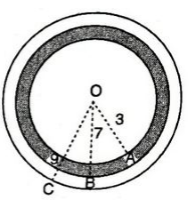Solution:

1st circle - with radius 3

2nd circle - with radius 7

3rd circle - with radius 9

Area of 1st circle = π(3)2 = 9π

Area of 2nd circle = π(7)2 = 49π

Area of 3rd circle = π(9)2 = 81π

Area of shaded region = Area of 2nd circle - Area of 1st circle

= 49π − 9π

= 40π

Probability that it will land on the shaded region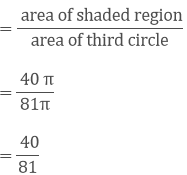Question: 4

In below fig. points A, B, C and D are the centers of four circles that each has a radius of length one unit. If a point is selected at random from the interior of square ABCD. What is the probability that the point will be chosen from the shaded region?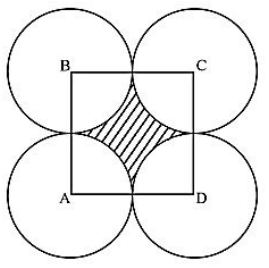Solution:

Radius of circle = 1 cm

Length of side of square = 1 + 1 = 2 cm

Area of square = 2 × 2 = 4 cm2

Area of shaded region = Area of a square - 4 × Area of the quadrant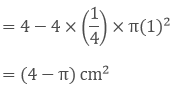Probability that the point will be chosen from the shaded region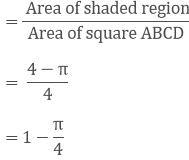Since geometrical probability,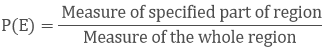Question: 5

In the fig. below, JKLM is a square with sides of length 6 units. Points A and B are the midpoints of sides KL and LM respectively. If a point is selected at random from the interior of the square. What is the probability that the point will be chosen from the interior of triangle JAB?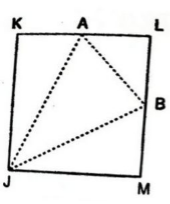Solution:

JKLM is a square with sides of length 6 units.

Points A and B are the midpoints of sides KL and ML, respectively.

If a point is selected at random from the interior of the square.

We have to find the probability that the point will be chosen from the interior of ΔJAB.

Now, Area of square JKLM is equal to 62 = 36 sq.units

Now, we have ar(ΔKAJ) = 1/2 × AK × KJ

= 1/2 × 3 × 6

= 9 unit2

ar(ΔJMB) = 1/2 × JM × BM

= 1/2 × 6 × 3

= 9 unit2

ar(ΔAJB) = 1/2 × AL × BL

=1/2 × 3 × 3

= 9/2 unit2

Now, an area of the triangle AJB ar(ΔAJB) = 3 × 9/2

= 27/2 unit2

We know that: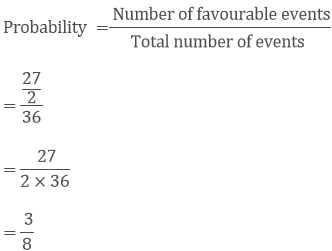Hence, the probability that the point will be chosen from the interior of ΔAJB = 3/8.

Question: 6

In the fig. below, a square dartboard is shown. The length of a side of the larger square is 1.5 times the length of a side of the smaller square. If a dart is thrown and lands on the larger square. What is the probability that it will land in the interior of the smaller square?Solution:

Let, the length of the side of smaller square = a

The a length of a side of bigger square = 1.5a

Area of smaller square = a2

Area of bigger square = (1.5)2a2 = 2.25a2

Probability that dart will land in the interior of the smaller squareGeometrical probability,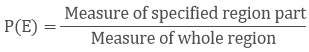```### Course Features

• 728 Video Lectures
• Revision Notes
• Previous Year Papers
• Mind Map
• Study Planner
• NCERT Solutions
• Discussion Forum
• Test paper with Video Solution